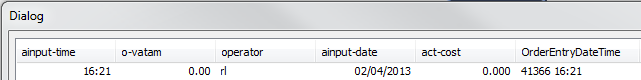# QlikView App Dev

Discussion Board for collaboration related to QlikView App Development.

Announcements
Join “Do More With Qlik” with Mike Tarallo to get a first-hand look at the New Authoring Experience, June 29th: REGISTER HERE
cancel
Showing results for
Did you mean:Not applicable

## Splitting down the day

I am loading a sales table that has separate date and time columns.

In my chart I want an expression that brings through the data based on the date AND time up to the minute of the current time.

e.g. if the time is 15:00 I want all the data up to and including this time on the date I have already specified below.

This is the expression I am using to identify the correct previous day (it is effectively the equivalent sales day from the previous year) but I need to also include the time criteria as above.

Sum({<[o-date]={'\$(=Date(Today()-363))'}>}[NetSales])

o-date is the date field  (yyyy-mm-dd)

NetSales is the sales value

ainput-time is the entry time (hh:mm)

11 RepliesMVP

Maybe

Sum({<[o-date]={'\$(=Date(Today()-363))'}, [ainput-time] = {"<=\$(=Time(Now(1)))"}>}[NetSales])

Check the timer modes (argument to time() ) for what is appropriate for your setting. The result of the dollar sign expansion should match the format of your ainput-time field, so you may need to adjust the format, too.MVP

Stefan, shouldn't we just add the formats here just to be safe here:

Sum({<[o-date]={"\$(=Date(Today()-363, 'YYYY-MM-DD'))"}, [ainput-time] = {"<=\$(=Time(Now(1), 'hh:mm'))"}>}[NetSales])

or make the following change in the script:

SET TimeFormat='hh:mm';

SET DateFormat='YYYY-MM-DD';

and then use the expression you have given as is

Sum({<[o-date]={'\$(=Date(Today()-363))'}, [ainput-time] = {"<=\$(=Time(Now(1)))"}>}[NetSales])Not applicable
Author

Thank you for the great replies, they worked well.

The results are not not what I expected (they are the correct figures though), I hadn't taken into account the input date.

Can you help expand the criteria of time please where the combination of:

[ainput-date] and [ainput-time] are before Now

Thanks for helpMaster III

It would be easy to filter if you have a timestamp may be like [ainput-datetime]..MVP

You can also do a similar search on ainput-date:

{<

[o-date]={'\$(=Date(Today()-363))'},

[ainput-time] = {"<=\$(=Time(Now(1)))"},

[ainput-date] = {"<=\$(=Date(now(1)))"}

>}Creator

Hi,

Create a new column in your data table containing the timestamp:

Timestamp(Timestamp#([o-date] &' ' & [ainput-time], 'yyyy-mm-dd hh:mm')) AS SalesTimestamp

Then, use this expression in your object to limit the data to sales from "now" and back:

Sum({<SalesTimestamp={"<=\$(=Now())"}>}[NetSales])

Hope this helps!Not applicable
Author

The [ainput-date] field is displaying in its own field as 02/04/2013 and [ainput-time] as 16:21

When using the line below the new column is displaying as 41366 16:21 and not 2013-04-02 16:21 as I need.

What as I doing wrong ?

Timestamp(Timestamp#([o-date] &' ' & [ainput-time], 'yyyy-mm-dd hh:mm')) AS SalesTimestampMVP

If date and time already show a numeric representation, it should be enough to write:

Timestamp( [o-date] + [ainput-time], 'yyyy-mm-dd hh:mm') AS SalesTimestampMaster III

hi sunindia/Swuehl

I have a doubt regarding the above expression..Would you mind clarifying it?

Sum({<[o-date]={'\$(=Date(Today()-363))'}, [ainput-time] = {"<=\$(=Time(Now(1)))"}>}[NetSales])

will the above expression filter records that are less than the current time for all the days? in other words, are the dates and times are filtered independently?